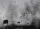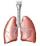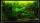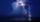# Brick wall

Garden 70 m long and 48 m wide should surround with wall 2.1 meters high and 30 cm thick. Wall will be built on the garden ground. How many will we need bricks if to 1 m³ is required approximately 300 bricks?

Result

n =  44378

#### Solution:Leave us a comment of example and its solution (i.e. if it is still somewhat unclear...):Math student
unprecise - need to know if wall needs to be build ON garden-ground (like in the solution) or if wall should enclose whole garden - in that case:
2.1 * ( 2 * 48 + 2 * 70 ) * 0.3 * 300 = 44604 does the trickDr Math
thank you, we corrected the wording of the problem. But your solutions 44604  tricks is also wrong because in the corners some bricks are not counted.#### To solve this example are needed these knowledge from mathematics:

Do you want to convert area units? Do you know the volume and unit volume, and want to convert volume units?

## Next similar examples:

1. RainfallOn Thursday, fell 1 cm rainfall. How many liters of water fell to rectangular garden with dimensions of 22 m x 35 m?
2. WaterIn the garden with an area of 8 ares rain 40hl of water. To what heights leveled water?
3. Water tank 2Water tank cuboid is 12 meters long and 6.5 meters wide and 1.2 meters high. How many hectoliters are in the tank when it is filled to 81%?
4. Concrete boxThe concrete box with walls thick 5 cm has the following external dimensions: length 1.4 m, width 38 cm and height 42 cm. How many liters of soil can fit if I fill it to the brim?
5. Cuboid aquariumCuboid 25 times 30 cm. How long is third side if cuboid contains 30 liters of water?
6. Water poolWhat water level is in the pool shaped cuboid with bottom dimensions of 25 m and 10 meters, when is 3750hl water in the pool.
7. DiggingA pit is dug in the shape of a cuboid with dimensions 10mX8mX3m. The earth taken out is spread evenly on a rectangular plot of land with dimensions 40m X 30m. What is the increase in the level of the plot ?
8. Water levelHow high reaches the water in the cylindrical barell with a diameter of 12 cm if there is a liter of water? Express in cm with an accuracy of 1 decimal place.
9. Water lakeThe length of the lake water is 8 meters width 7 meters and depth 120 centimeters. How many liters of water can fit into the water lake?
10. Swimming pool 4The pool shaped cuboid measuring 12.5 m × 640 cm at the bottom is 960hl water. To what height in meters reaches the water level?
11. RainfallThe annual average rainfall in India was in Cherrapunji in the year 1981 26 461 mm. How many hectoliters of water fell on 1 m2? Would fit this amount of water into a cube of three meters?
12. SportsmanA trained athlete is able to exhale after a deep breath still 500 ml of air. At normal inhalation and exhalation is breathing 500 ml of air. Within one minute, one breath and exhaled 14 times. What part of breathing air per day is one exhalation?
13. Reservoir 3How many cubic feet of water is stored in the reservoir that has a length of 200 feet, a width of 100 feet, an overflow depth of 32 feet, and a current water level of 24 feet?
14. Water tankWater tank shape cuboid has a width of 3.1 m and length twice larger. How high will reach water if water flow into 13 liters of water per second during 16 minutes?
15. FlowerbedThe flowerbed has a length 3500mm and a width 1400mm. How many foil is needed to covers the flowerbed? How many m2 of foil was consumed for its production (add 10% of the material to the joint and waste)? How many liters of air is inside the enclosure? (F
16. WaterOn the lawn which has area 914 m2 rained 3 mm of water. How many liters of water rained?
17. TrayWjat height reach water level in the tray shaped a cuboid, if it is 420 liters of water and bottom dimensions are 120 cm and 70 cm.